﻿ 自动修井机液压大钳液压控制系统的设计研究

自动修井机液压大钳液压控制系统的设计研究Research on Hydraulic Control System of the Hydraulic Tong for Automatic Workover Rig

Abstract: At present, the difficulty for workover rig is low degree of automation in China. However, the cost of designing a new automatic workover rig is so high that it is not suitable for the situation of low oil prices, so we need to upgrade the current workover rig to the automatic one. For automatic workover rig, its tubing and rod thread screw on or off automatically is a key. The hydraulic tong, which applied to the automatic workover rig, is designed. According to its function and the process of action, we also design the hydraulic control system. For two kinds of hydraulic motors, one for elevating and the other for thread screwing on or off, we carry out their parameter calculation and selection. Likewise, we use AMESim to simulate the hydraulic control system of hydraulic tong and the hydraulic system for thread screwing on or off. The simulation results are consistent with the design of action.

1. 引言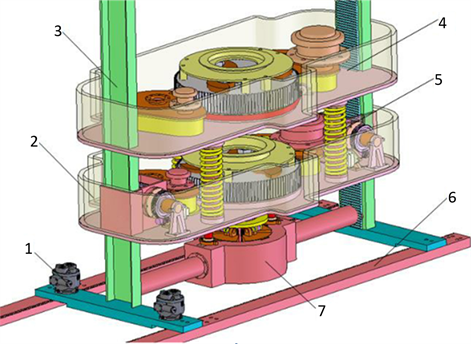1.水平运移马达；2.背钳；3.升降导轨；4.主钳；5.间隙调节装置；6.底座；7.动力卡瓦

Figure 1. Structure of closed hydraulic pliers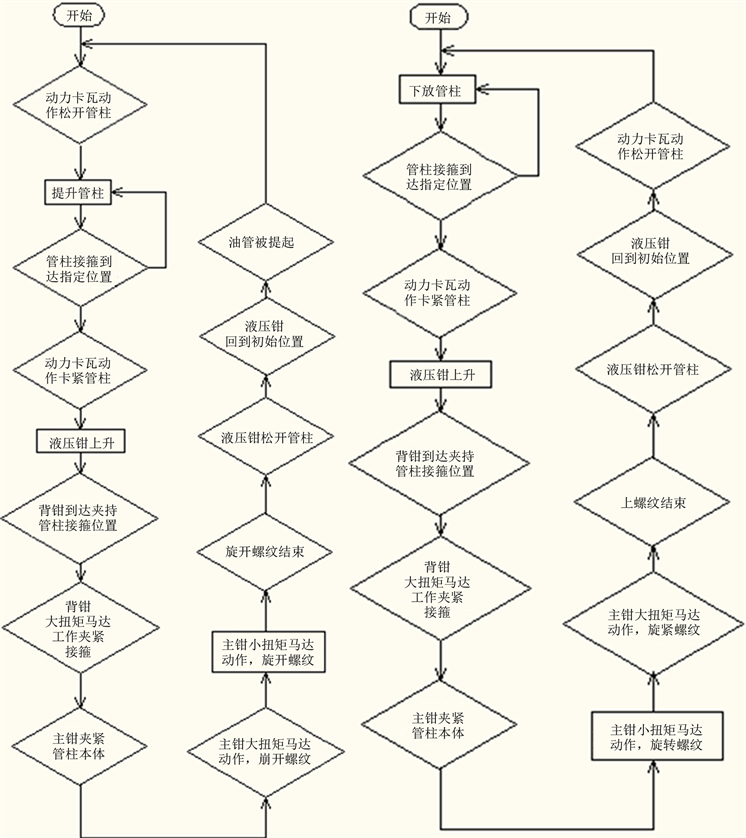(a) 卸螺纹动作流程 (b) 上螺纹动作流程

2. 自动修井机液压大钳液压控制系统设计

2.1. 动力卡瓦夹紧-松开回路

2.2. 液压大钳升降回路

2.3. 液压大钳上卸螺纹回路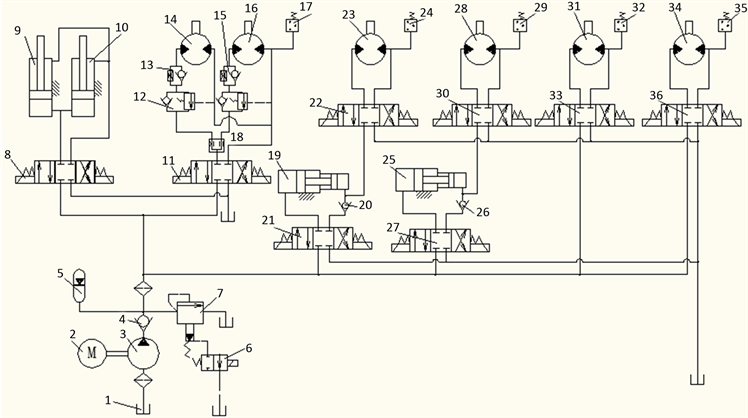1.油箱；2.电动机；3.液压泵；4、20、26.单向阀；5.蓄能器；6.二位二通电磁阀；7.先导式溢流阀；8、11、21、22、27、30、33、36.三位四通电磁阀；17、24、29、32、35.压力开关；9、10.动力卡瓦液压缸；12.平衡阀；13、15.单向节流阀；14、16.升降液压马达；18.分流集流阀；19、25.增压缸；23.主钳大扭矩马达；28.背钳大扭矩马达；31.主钳小扭矩马达；34.背钳小扭矩马达

Figure 3. The hydraulic system of automatic hydraulic pliers

3. 自动修井机液压钳液压马达的参数计算

3.1. 升降液压马达的参数计算

${n}_{1}=\frac{\upsilon }{2\pi r}=\frac{0.25}{2\pi ×0.09}=26.34\text{\hspace{0.17em}}\text{r}/\mathrm{min}$

${T}_{1}=\frac{mgd}{4}=\frac{5057×9.8×0.18}{4}=2230.5\text{\hspace{0.17em}}\text{N}\cdot \text{m}$

$n=i{n}_{1}=26.34×62=1633.08\text{\hspace{0.17em}}\text{r}/\mathrm{min}$

$q=\frac{2\pi T}{p}=\frac{2\pi ×51.3}{16}=20.1\text{\hspace{0.17em}}\text{mL}/\text{r}$

$Q=\frac{qn}{1000}=\frac{20.1×1633.08}{1000}=32.8\text{\hspace{0.17em}}\text{L}/\mathrm{min}$Table 1. Parameters of worm gear and wormTable 2. The parameters and model of the lifting hydraulic motor

3.2. 主、背钳液压马达的参数确定

1) 主钳大扭矩马达的参数计算

$T=\frac{{T}_{1}}{i\eta }=\frac{8000}{5×0.94}=1702.13\text{\hspace{0.17em}}\text{N}\cdot \text{m}$

$q=\frac{2\pi T}{p}=\frac{2\pi ×1702.13}{20}=534.47\text{\hspace{0.17em}}\text{mL}/\text{r}$

2) 主钳小扭矩马达的参数计算

$T=\frac{{T}_{1}}{i\eta }=\frac{2300}{5×0.94}=489.36\text{\hspace{0.17em}}\text{N}\cdot \text{m}$

4. 液压控制系统的仿真分析Table 3. Parameters of high torque motor

4.1. 液压钳升降回路仿真分析

4.2. 液压大钳上卸螺纹回路仿真分析Table 4. Parameters of small torque motor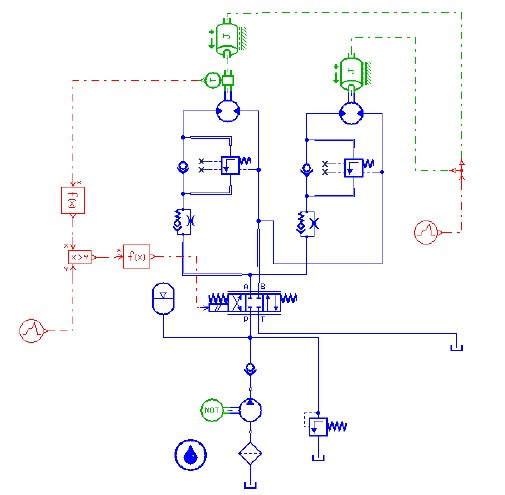Figure 4. The simulation model of hydraulic clamp lift circuit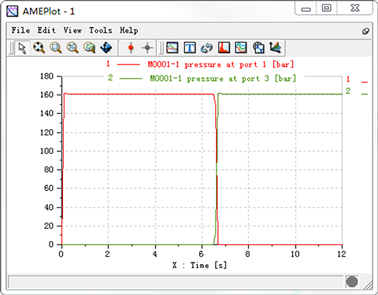Figure 5. The inlet pressure-time curve of lifting motor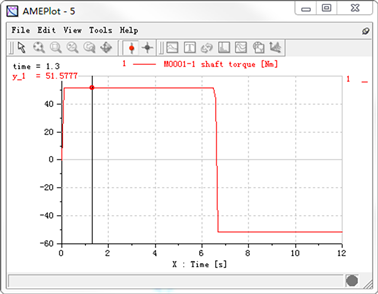Figure 6. Torque-time curve of lifting motor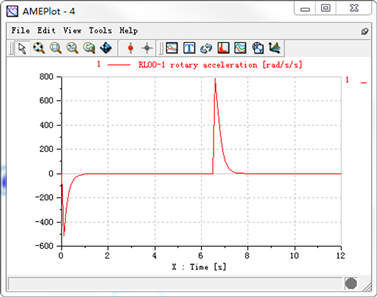Figure 7. Angular acceleration-time curve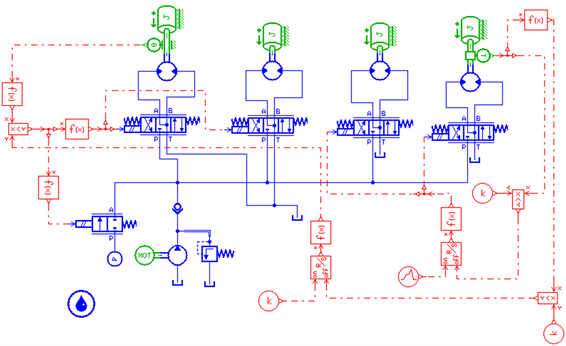Figure 8. The simulation model of shackle system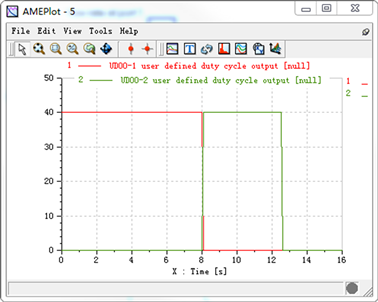Figure 9. The electromagnetic valve actuation signal-time curve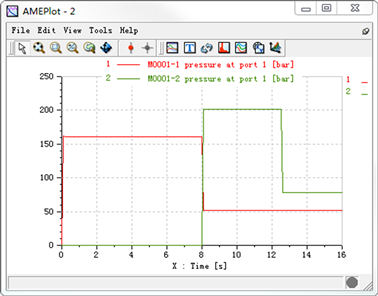Figure 10. Motor oil inlet pressure-time curve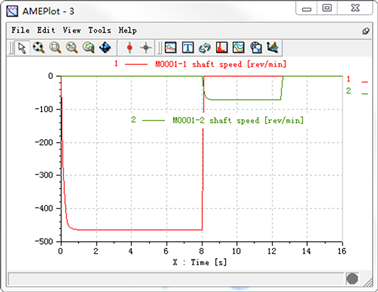Figure 11. Motor speed-time curve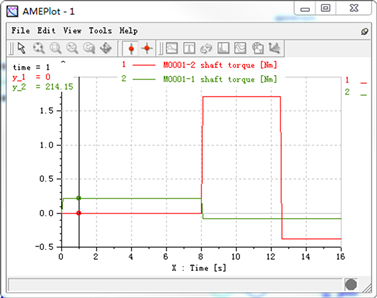Figure 12. Tightly motor torque-time curve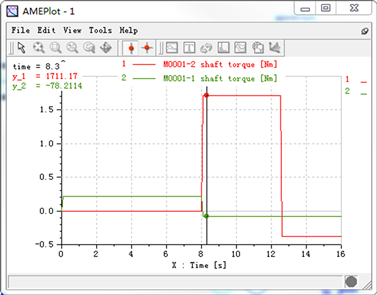Figure 13. Spinner motor torque-time curve

5. 结论

1) 设计的液压控制回路能够实现主、背钳升降以及螺纹副的上卸螺纹和紧松螺纹的功能。

2) 利用AMESim软件对主、背钳升降液压控制系统和螺纹副的上卸螺纹液压系统进行了仿真，仿真结果与计算结果相符，说明液压系统的性能达到设计要求。

 常玉连, 肖易萍, 高胜. 修井井口机械化自动化装置的研究进展[J]. 石油矿场机械, 2008, 37(5): 62-67.

 高盛, 庞伶伶. 修井井口机械自动化技术现状分析与展望[J]. 石油机械, 2012(2): 80-85.

 耿玉广, 谷全福, 王树义, 等. 修井作业井口无人操作起下油管装置[J]. 石油钻采工艺, 2014(6): 116-121.

 朱小明. 单向顺序阀和平衡阀的区别——兼对《平衡阀和双向液压锁的正确选用》一文的异议[J]. 建筑机械, 2007(11s): 88-89.

 成大先. 机械传动[M]. 上海: 化学工业出版社, 2013: 575-589.

 Palm, W.J. (1999) Modeling, Analysis and Control of Dynamic Systems. 2nd Edition, John Wiley & Sons, Inc., New York.

 Karnopp, D.C., Margolis, D.L. and Rosen-berg, R.C. (2000) Systems Dynamics: Modeling and Simulation of Mechatronics Systems. 3rd Edition, John Wiley & Sons, Inc., New York.

 Louca, L.S., Stein, J.L. and Hulbert, G.M. (1998) A Physical-Based Model Reduction Metric with an Application to Vehicle Dynamics. IFAC Proceedings Volumes, 31, 585-590.

Top